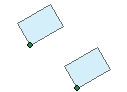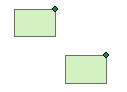Open Table of Contents

Points To Rectangles

Go to ToolBox Implementation

Creates rectangles from points in a point dataset and user defined width, height rotation angle and location of the point.

Inputs:

• A Point feature class
• Rectangle Width. The width can be fixed for all points or different assigned from the values in a numeric field of the point attribute table
• Rectangle Height. The height can be fixed for all points or different assigned from the values in a numeric field of the point attribute table
• Rotation angle. The angle can be fixed for all points or different assigned from the values in a numeric field of the point attribute table
• Point location. This parameter defines what will be the location of the resulting rectangles in relation to the original points.

Outputs:

• New polygon feature class. All the original field values will be transferred from the points to the polygons.

Notes:

• The values for the Width and the Height should be in the units of the spatial reference of the input Point dataset
• The angle (if used) should be in Decimal Degrees and have Polar orientation - East = 0, anti-clockwiseExamples:

 Width = 300, Height = 200 Angle = 15, Location = "Center" Width = 300, Height = 200 Angle = 15, Location = "LL" (Lower Left) Width = 300, Height = 200 Angle = 0, Location = "UR" (Upper Right)Command line syntax

ET_GPPointsToRectangles <input_dataset> <out_feature_class> <width_field> <height_field> <angle_field> <LowerLeft | UpperLeft | UpperRight | LowerRight | Center>

Parameters

Expression Explanation
<input_dataset> A Point feature class or feature layer
<out_feature_class> A String - the full name of the output feature class (A feature class with the same full name should not exist)
<width_field> A String representing the name of a field in the in the attribute table of the input dataset field name.  The field has the values for the width of the rectangles to be created.
<height_field> A String representing the name of a field in the in the attribute table of the input dataset field name.  The field has the values for the height of the rectangles to be created.
<angle_field> A String representing the name of a field in the in the attribute table of the input dataset field name.  The field has the values for the rotation angle of the rectangles to be created. The angle should be in Decimal Degrees and have Polar orientation - East = 0, anti-clockwise.
<LowerLeft | UpperLeft | UpperRight | LowerRight | Center> Required. A String - This parameter defines what will be the location of the resulting rectangles in relation to the original points.. The available options are (Case sensitive):
• "LL"  - Lower Left corner of the rectangles will be located on the input point.
• "LR"  - Lower Right corner of the rectangles will be located on the input point.
• "UL"  - Upper Left corner of the rectangles will be located on the input point.
• "UR"  - Upper Right corner of the rectangles will be located on the input point.
• Any other string used will cause the centers of the rectangles to be located on the input point.

Scripting syntax

ET_GPPointsToRectangles (input_dataset, out_feature_class,width_field,height_field, angle_field, location_type)

See the explanations above:
<> - required parameter
{} - optional parameter